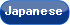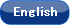Please use this identifier to cite or link to this item: `http://hdl.handle.net/10119/4893`

 Title: A Unified Scheme for Detecting Fundamental Curves in Binary Edge Images Authors: Asano, TetsuoKatoh, NaokiTokuyama, Takeshi Keywords: AlgorithmDigital CurveArrangementImage Recognition Issue Date: 2001-03 Publisher: Elsevier Magazine name: Computational Geometry Volume: 18 Number: 2 Start page: 73 End page: 93 DOI: 10.1016/S0925-7721(01)00002-5 Abstract: We present a unified scheme for detecting digital components of various planar curves in a binary edge image. A digital component of a curve is the set of input edge points from each of which the horizontal or vertical distance to the curve is at most 0.5. Our algorithm outputs all curve components containing at least k points (k is a given threshold) in O(n^d) time (if d ≥ 2) and linear space, where n is the number of points, and d is a measure that reflects the complexity of a family of curves; for example, d = 2, 3, and 5 for lines, circles, and ellipses, respectively. For most of the popular families of curves, our only primitive operations are algebraic operations of bounded degree and comparisons. We also propose an approximate algorithm for computing an approximation solution with error ratio ε = 1 - α (called an α-sensitive solution), whose time complexity is O((t/ε)^n), which is very efficient if the threshold-ratio t = n/k is small. Rights: NOTICE: This is the author's version of a work accepted for publication by Elsevier. Tetsuo Asano, Naoki Katoh and Takeshi Tokuyama, Computational Geometry, 18(2), 2001, 73-93, http://dx.doi.org/10.1016/S0925-7721(01)00002-5 URI: http://hdl.handle.net/10119/4893 Material Type: author Appears in Collections: b10-1. 雑誌掲載論文 (Journal Articles)

Files in This Item:

File Description SizeFormat